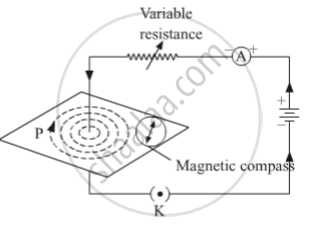# Draw Magnetic Field Lines Produced Around a Current Carrying Straight Conductor Passing Through Cardboard. Name, State and Apply the Rule to Mark the Direction of These Field Lines. - Science

Draw magnetic field lines produced around a current-carrying straight conductor passing through cardboard. Name, state and apply the rule to mark the direction of these field lines.

#### SolutionTo mark the direction of magnetic field lines, we will use The Right-hand thumb rule. The right-hand thumb rule is used to find the direction of the magnetic field lines, according to this rule if we place our right-hand thumb along the direction of the current flowing in a current-carrying wire, the direction in which the fingers wrap the wire represents the direction of the magnetic field. As we can see in the given figure, where the current is going downward, the direction of magnetic field lines is clockwise, according to the rule.

Concept: Magnetic Field Lines
Is there an error in this question or solution?